# Fractions - math word problems

1. Alcohol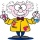6l 48% alcohol, how much 52% alcohol must be added to give 50% alcohol?
2. A baker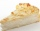A baker has 5 1/4 pies in her shop. She cut the pies in pieces that are each 1/8 of a whole pie. How many pieces of pie does she have?
3. Barrel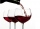Barrel of wine is filled by 9/14. What part of the barrel will remain filled when 1/4 of the wine we pour from the barrel?
4. Two workers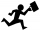One worker needs 40 hours to do a job, and the second would do it in 30 hours. They worked together for several hours, then the second was recalled, and the first completed the job itself in 5 hours. How many hours did they work together, and how much didWhy does 1 3/4 + 2 9/10 equal 4.65? How do you solve this?
6. Discount and pricesAfter a 29% discount, the new product price was € 426. What was the original price of the product?
7. The sum 4The sum of Robin's age is 45. Seven years ago, Robin was 16 years more than one half as old as Bruno then. How old is Bruno?
8. In centerWhat number lies in the center between a quarter of a fifth and a half of a third on the number axis?
9. Three-day tripThe cyclist on three-day trip travel 30% of the total route on the first day, 3/5 of the rest on the second day and 35 km on the third day. How many kilometers did travel cyclists each day and how many?
10. Video gameNicole is playing a video game where each round lasts 7/12 of an hour. She has scheduled 3 3/4 hours to play the game. How many rounds can Nicole play?
11. Three glasses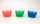Three glasses of different colors have different volumes. Red 1.5 liter is filled from 2/5, blue 3/4 liter is filled from 1/3, and the third green 1.2 liter is empty. Pour green glass 1/4 of the contents from the red glass and 2/5 of the content from the
12. New bridge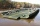Thanks to the new bridge, the road between A and B has been cut to one third and is now 10km long. How much did the road between A and B measure before?
13. Pizza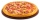Five friends were together for pizza. Adam divided his pizza into thirds, Boris in quarters, Denis in patina and Luke in sixth. Then Simon also came to them. Each of the five boys gave him one piece, leaving him one whole pizza. In how many equal parts di
14. ExpressionsLet k represent an unknown number, express the following expressions: 1. The sum of the number n and two 2. The quotient of the number n and nine 3. Twice the number n 4. The difference between nine and the number n 5. Nine less than the number n
15. Expressions with variableThis is algebra. Let n represent an unknown number and write the following expressions: 1. 4 times the sum of 7 and the number x 2. 4 times 7 plus the number x 3. 7 less than the product of 4 and the number x 4. 7 times the quantity 4 more than the n
16. Algebra problemThis is algebra. Let n represent an unknown number. 1. Eight more than the number n 2. Three times the number n 3. The product of the number n and eight 4. Three less than the number n 5. Three decreased by the number n
17. Two pen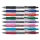Two pen and one notebook costs \$56. One pen costs 1/5 of one notebook. How much is the one notebook?
18. AlmondsRudi has 4 cups of almonds. His trail mix recipe calls for 2/3 cup of almonds. How many batches of trail mix can he make?
19. Turtles 2A box turtle hibernates in the sand at 11 5/8. A spotted turtle hibernates at 11 16/25 feet. Which turtle is deeper?
20. Tank of fuelA 14.5-gallon tank of fuel is 3/4 full. How many more gallons will it take to fill up the tank?

Do you have an interesting mathematical word problem that you can't solve it? Submit math problem, and we can try to solve it.

We will send a solution to your e-mail address. Solved examples are also published here. Please enter the e-mail correctly and check whether you don't have a full mailbox.

Please do not submit problems from current active competitions such as Mathematical Olympiad, correspondence seminars etc...

Need help calculate sum, simplify or multiply fractions? Try our fraction calculator.